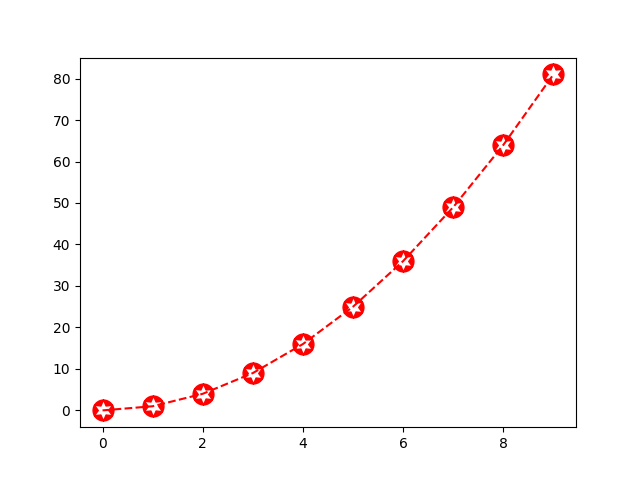# Marker Path¶

Using a Path as marker for a plot.

import matplotlib.pyplot as plt
import matplotlib.path as mpath
import numpy as np

star = mpath.Path.unit_regular_star(6)
circle = mpath.Path.unit_circle()
# concatenate the circle with an internal cutout of the star
verts = np.concatenate([circle.vertices, star.vertices[::-1, ...]])
codes = np.concatenate([circle.codes, star.codes])
cut_star = mpath.Path(verts, codes)

plt.plot(np.arange(10)**2, '--r', marker=cut_star, markersize=15)

plt.show()